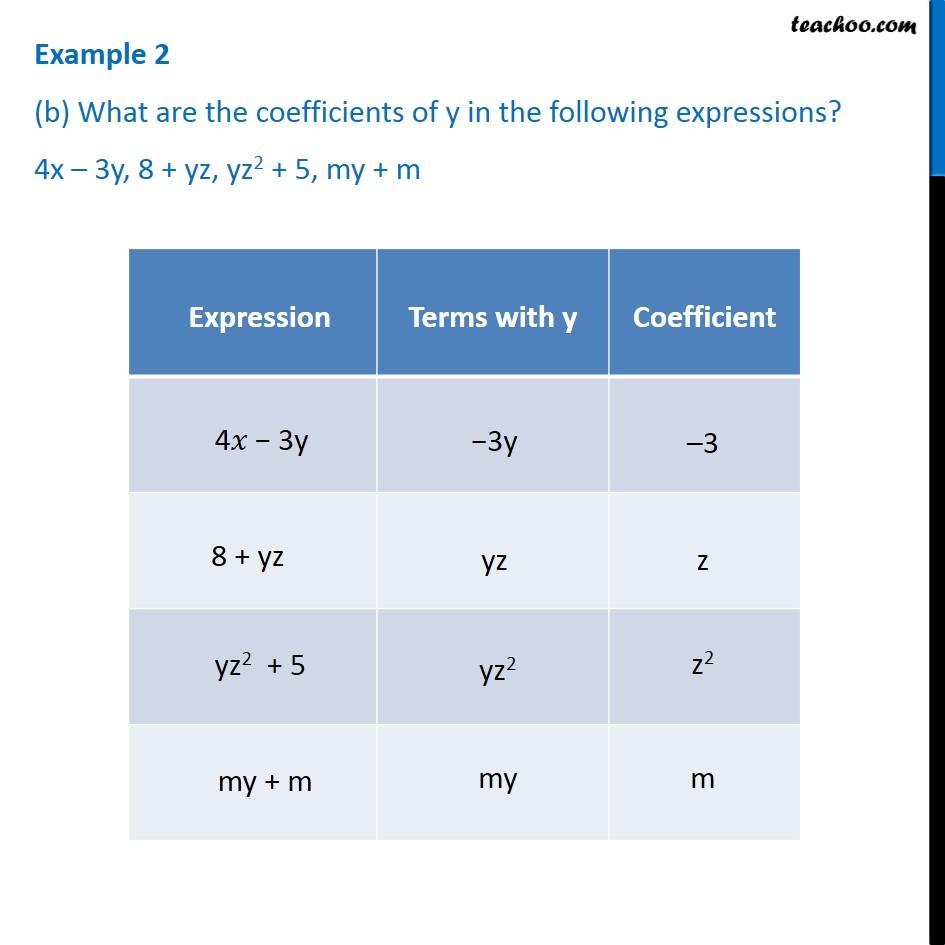Examples

Chapter 12 Class 7 Algebraic Expressions
Serial order wise### Transcript

Example 2 (b) What are the coefficients of y in the following expressions? 4x – 3y, 8 + yz, yz2 + 5, my + m Expression Terms with x Coefficient 4𝑥 − 3y −3y −3 8 + yz yz z yz2 + 5 −yz2 𝑥 z2 my + m my m